Electron. J. Diff. Equ., Vol. 2014 (2014), No. 207, pp. 1-29.

### Multiple solutions to asymmetric semilinear elliptic problems via Morse theory Leandro Recova, Adolfo Rumbos

Abstract:
In this article we study the existence of solutions to the problem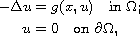where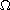is a smooth bounded domain in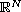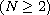and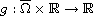is a differentiable function with g(x,0)=0 for all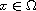. By using minimax methods and Morse theory, we prove the existence of at least three nontrivial solutions for the case in which an asymmetric condition on the nonlinearity g is assumed. The first two nontrivial solutions are obtained by employing a cutoff technique used by Chang et al in . For the existence of the third nontrivial solution, first we compute the critical group at infinity of the associated functional by using a technique used by Liu and Shaoping in . The final result is obtained by using a standard argument involving the Morse relation.

Submitted February 28, 2014. Published October 7, 2014.
Math Subject Classifications: 35J20.
Key Words: Morse theory; critical groups, local linking.

Show me the PDF file (372 KB), TEX file, and other files for this article.Leandro L. Recova Institute of Mathematical Sciences Claremont Graduate University Claremont, California 91711, USA email: leandro.recova@cgu.edu Adolfo J. Rumbos Department of Mathematics, Pomona College Claremont, California 91711, USA email: arumbos@pomona.edu